# NCERT Exemplar Class 11 Chemistry Solutions for Chapter 1 - Some Basic Concepts Of Chemistry Solutions

NCERT exemplar chemistry class 11 Chapter 1 Some Basic Concepts of Chemistry is essential study material for students to score good marks in class 11 and entrance examinations. Students are advised to get well versed with this exemplar solutions.

NCERT exemplar chemistry class 11 Chapter 1 has important questions with answers, class 11 chemistry chapter 1 exercise solution, class 11 chemistry chapter 1 numericals, MCQ’s HOTS, worksheets and questions from previous year question papers and sample papers.

Chemistry is one of the interesting science subjects that students can have fun with. However, this subject is not always easy and can be a bit confusing due to the introduction of a lot of new concepts, theories, equations, formulas and more. While each topic in chemistry advances from one level to another, in chapter 1 of class 11 chemistry textbook students will mainly study the development and importance of chemistry. To facilitate easy learning and to help students in getting a clear idea about the topics given in this chapter, we are offering free NCERT Exemplar for class 11 Chemistry chapter 1 – some basic concepts of chemistry here. The exemplar has been prepared by experts and provides answers to all the questions based on topics like matter, molecular and atomic mass, laws of chemical composition and more. The exemplar will not only help students understand all the topics clearly but they will be able to get an insight into all the important topics or questions .

Important topics of Chemistry chapter 1 Some Basic Concepts of Chemistry

1. Importance Of Chemistry
2. Nature Of Matter
3. Properties Of Matter And Their Measurement
• The International System Of Units (Si)
• Mass And Weight
4. Uncertainty In Measurement
• Scientific Notation
• Significant Figures
• Dimensional Analysis
5. Laws Of Chemical Combinations
• Law Of Conservation Of Mass
• Law Of Definite Proportions
• Law Of Multiple Proportions
• Gay Lussac’s Law Of Gaseous Volumes
6. Dalton’s Atomic Theory
7. Atomic And Molecular Masses
• Atomic Mass
• Average Atomic Mass
• Molecular Mass
• Formula Mass
8. Mole Concept And Molar Masses
9. Percentage Composition
10. Empirical Formula For Molecular Formula
11. Stoichiometry And Stoichiometric Calculations
• Limiting Reagent
• Reactions In Solutions.

Class 11 chemistry chapter 1 NCERT solutions can be accessed below: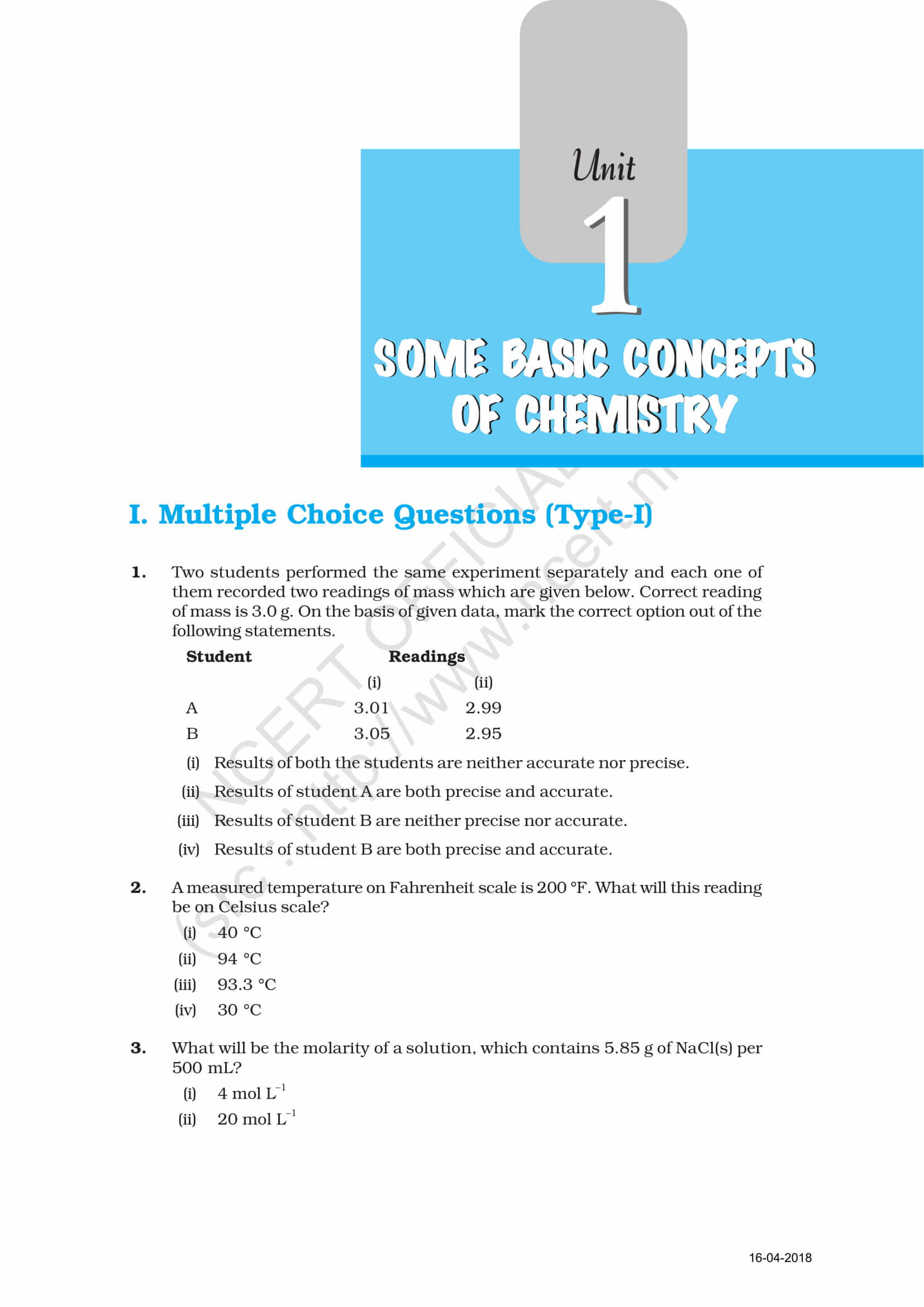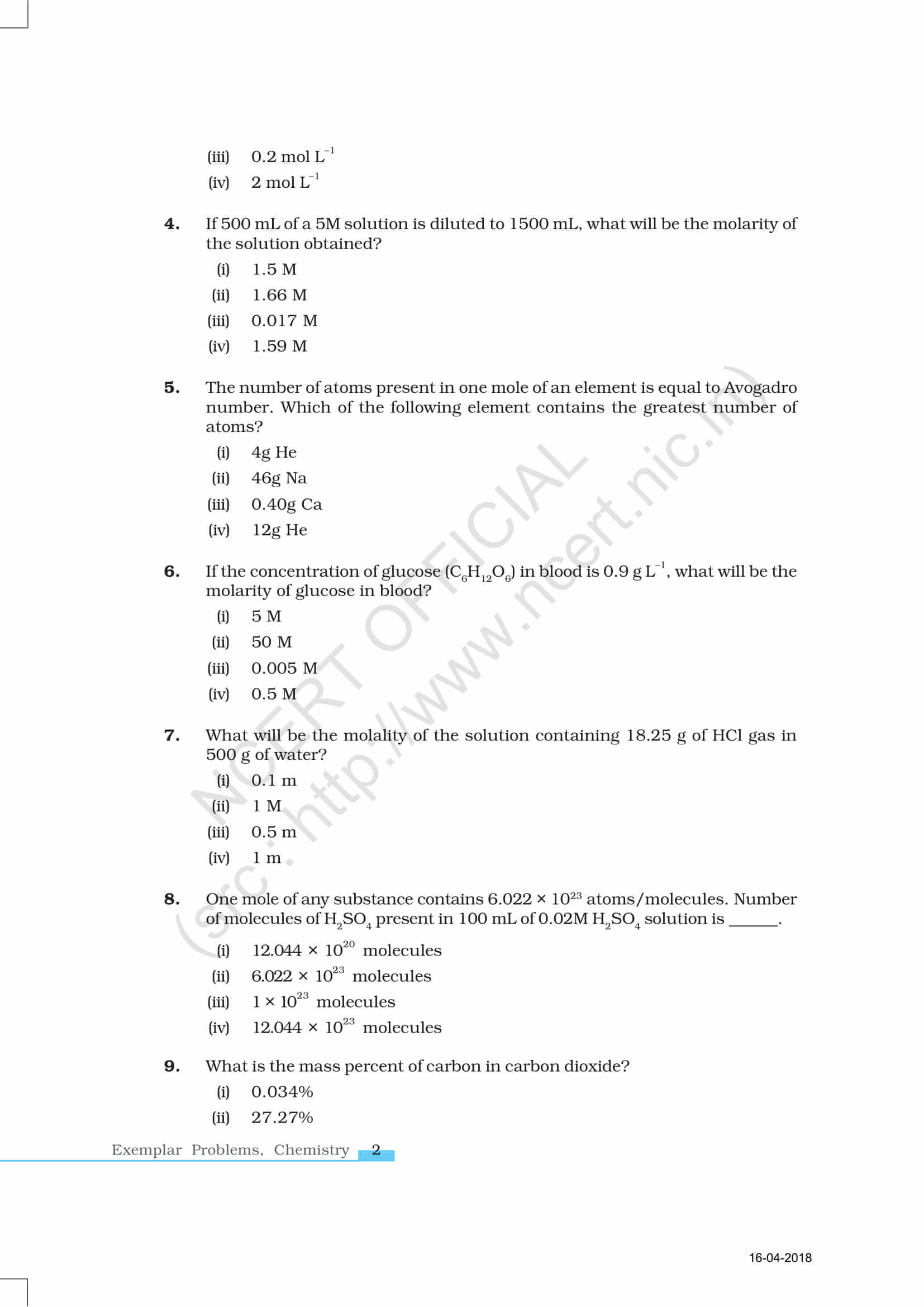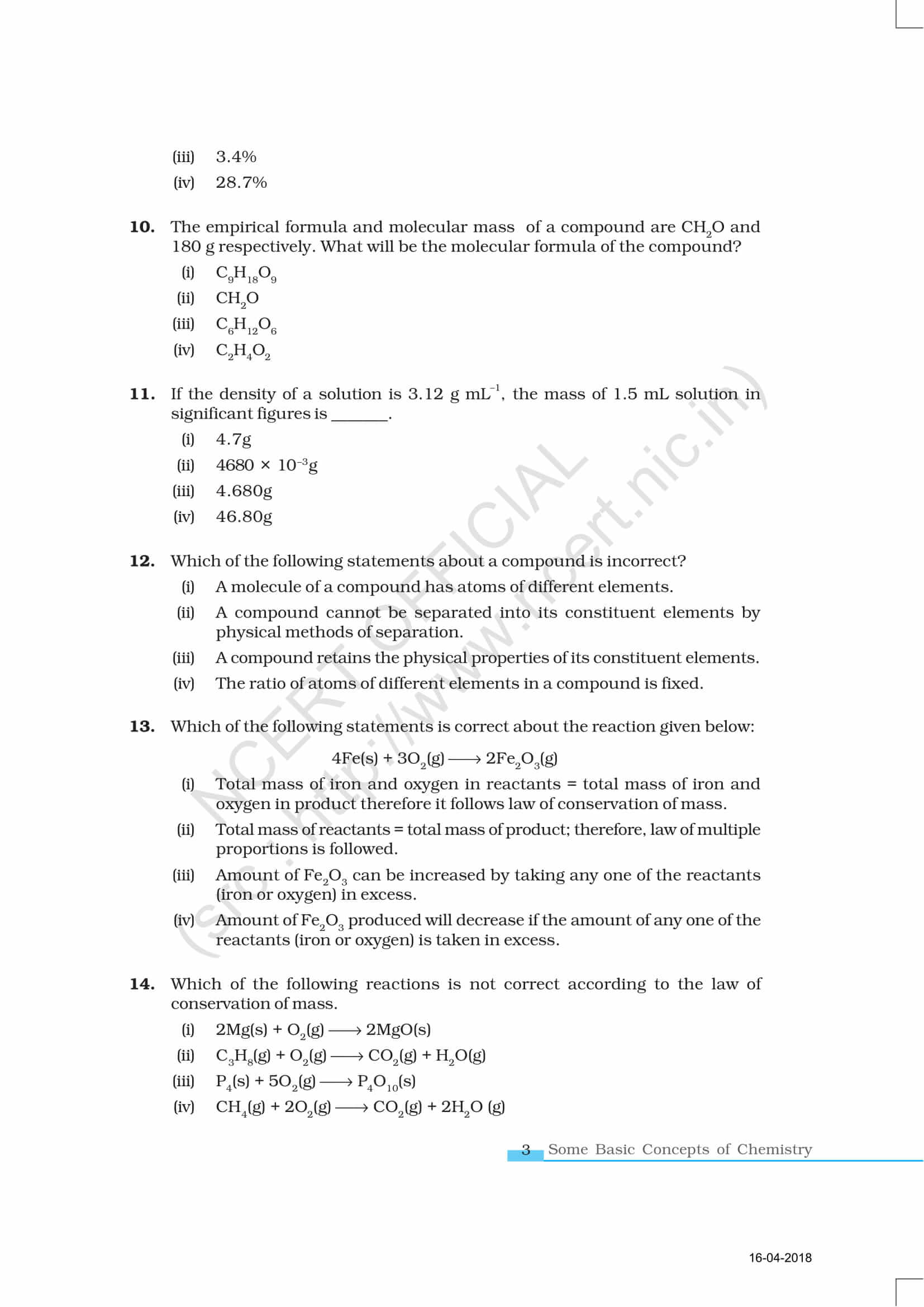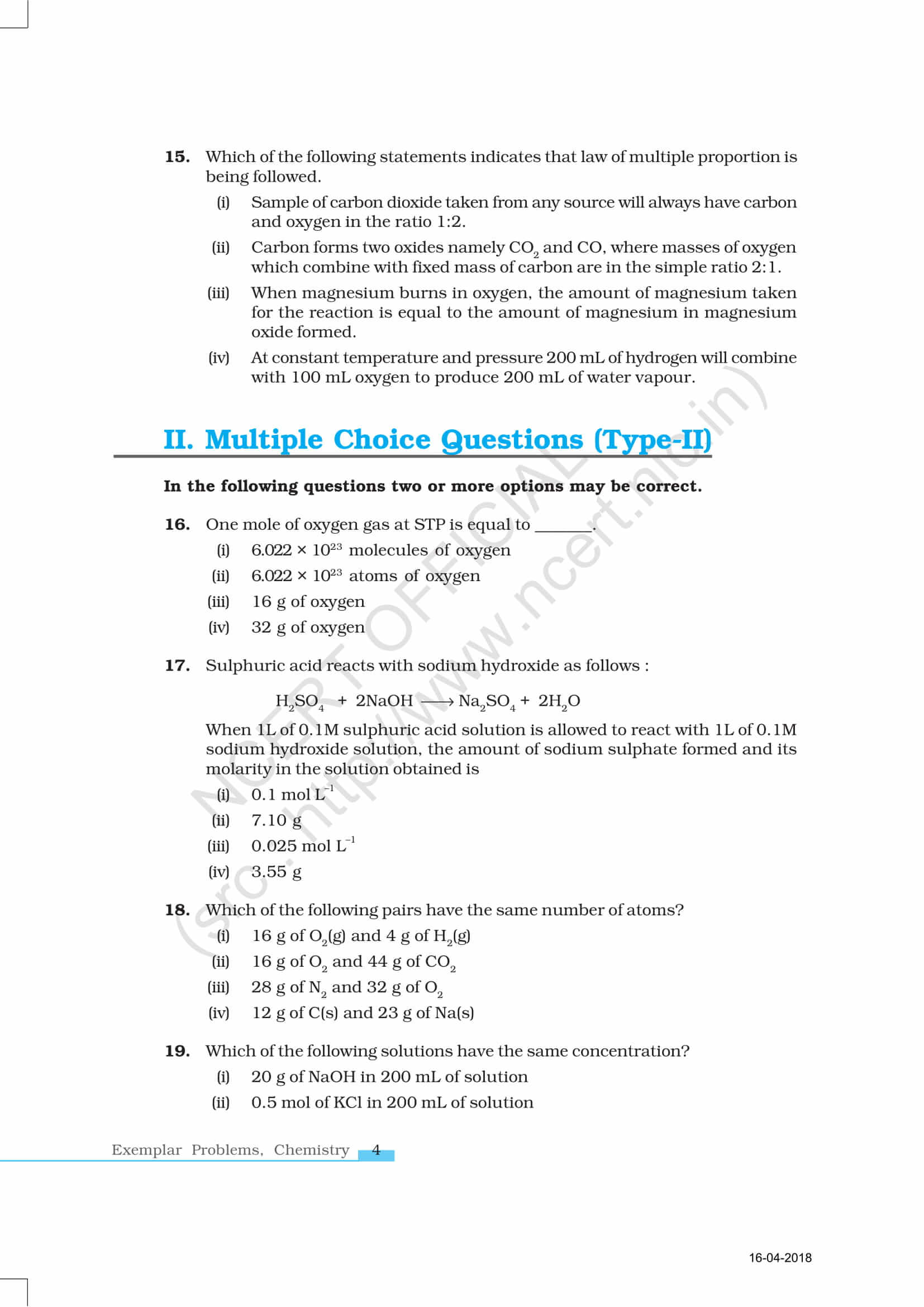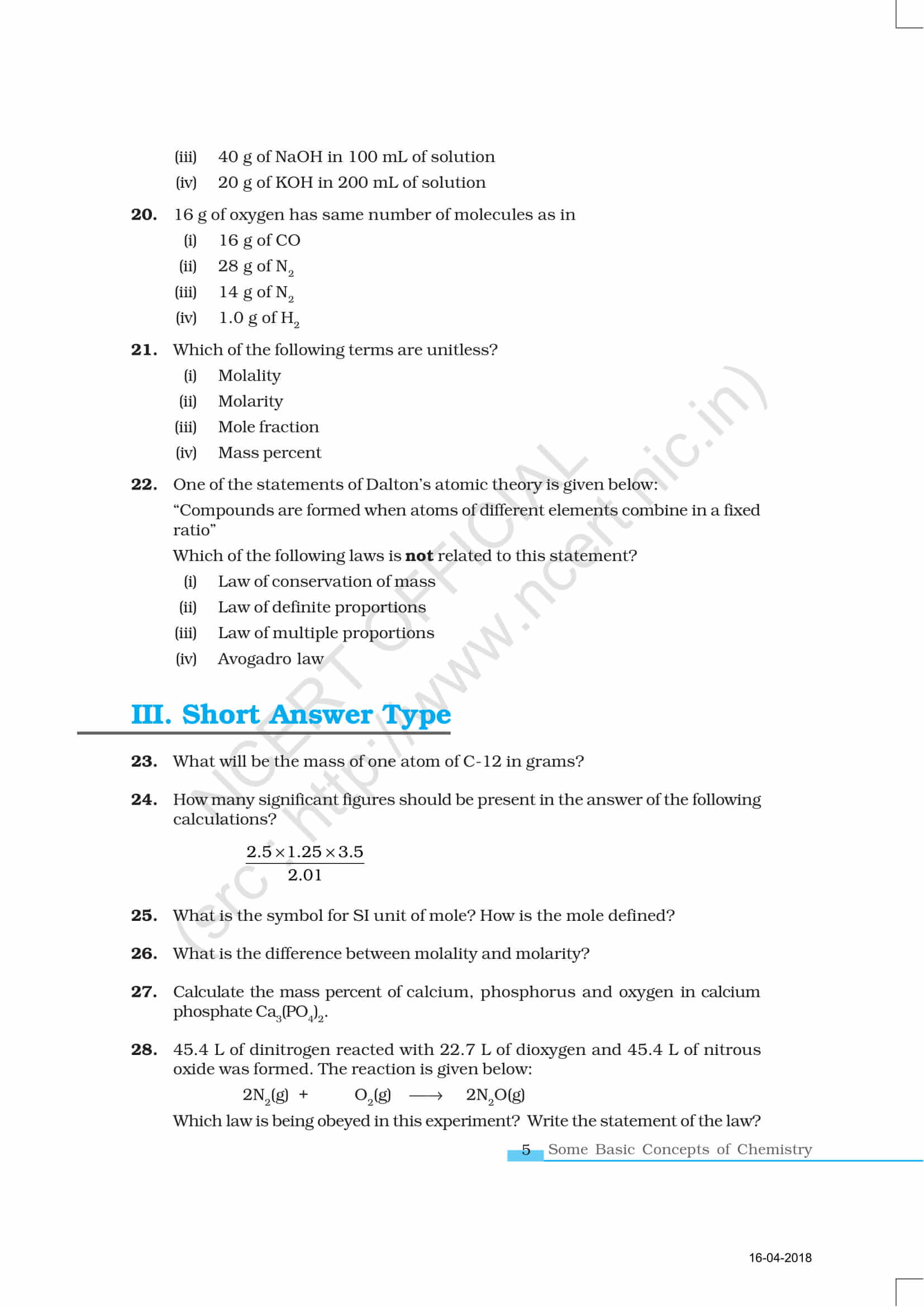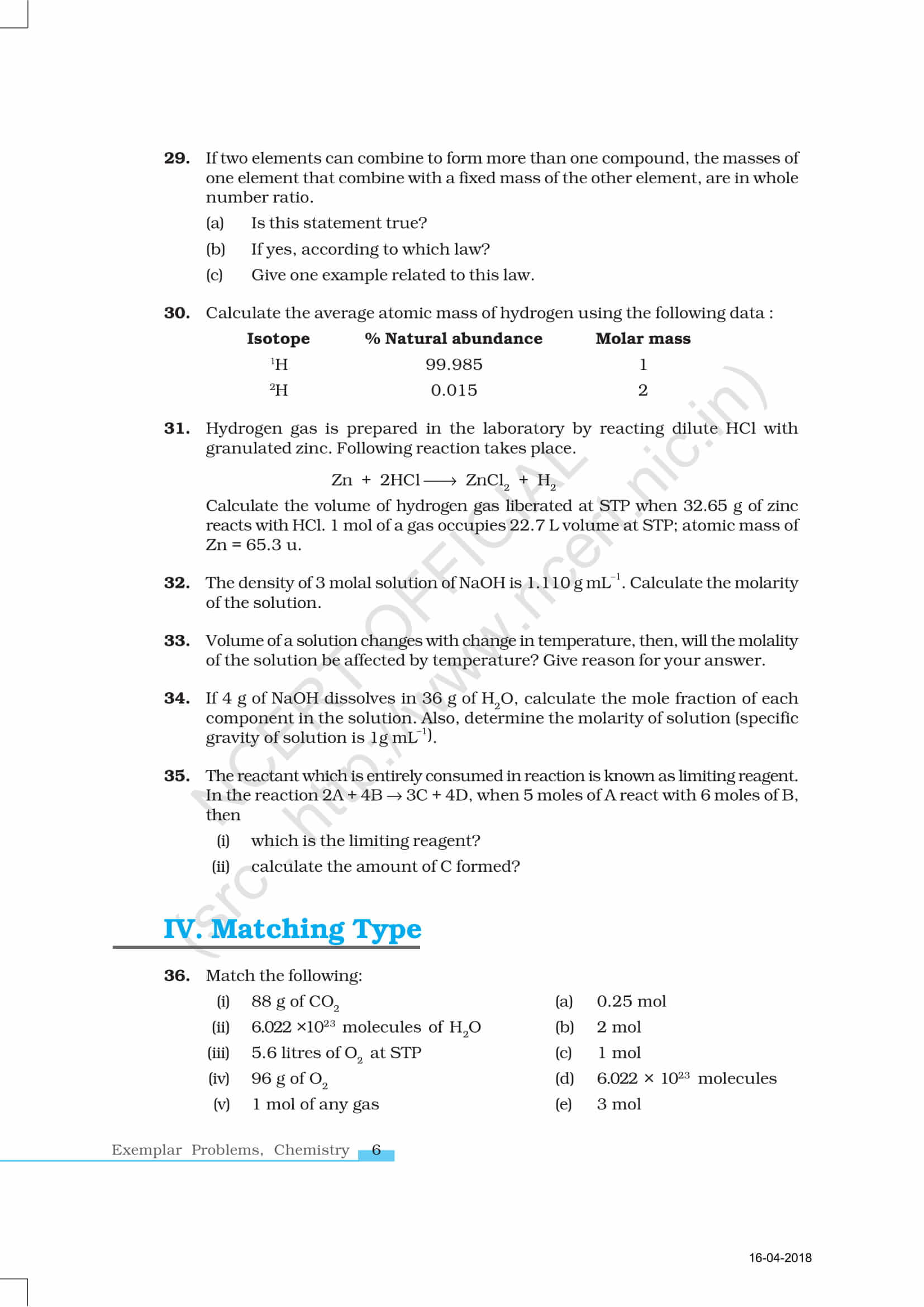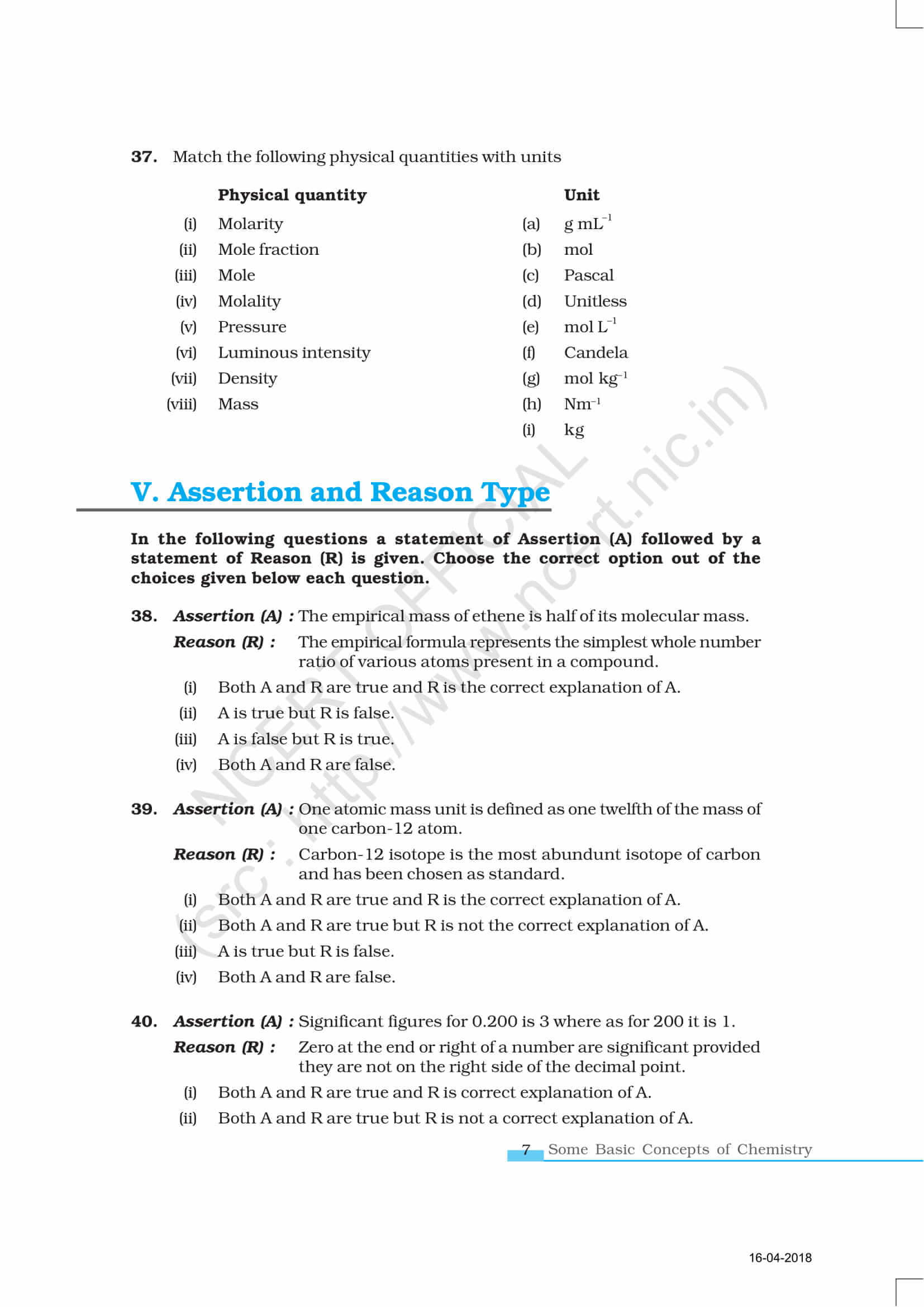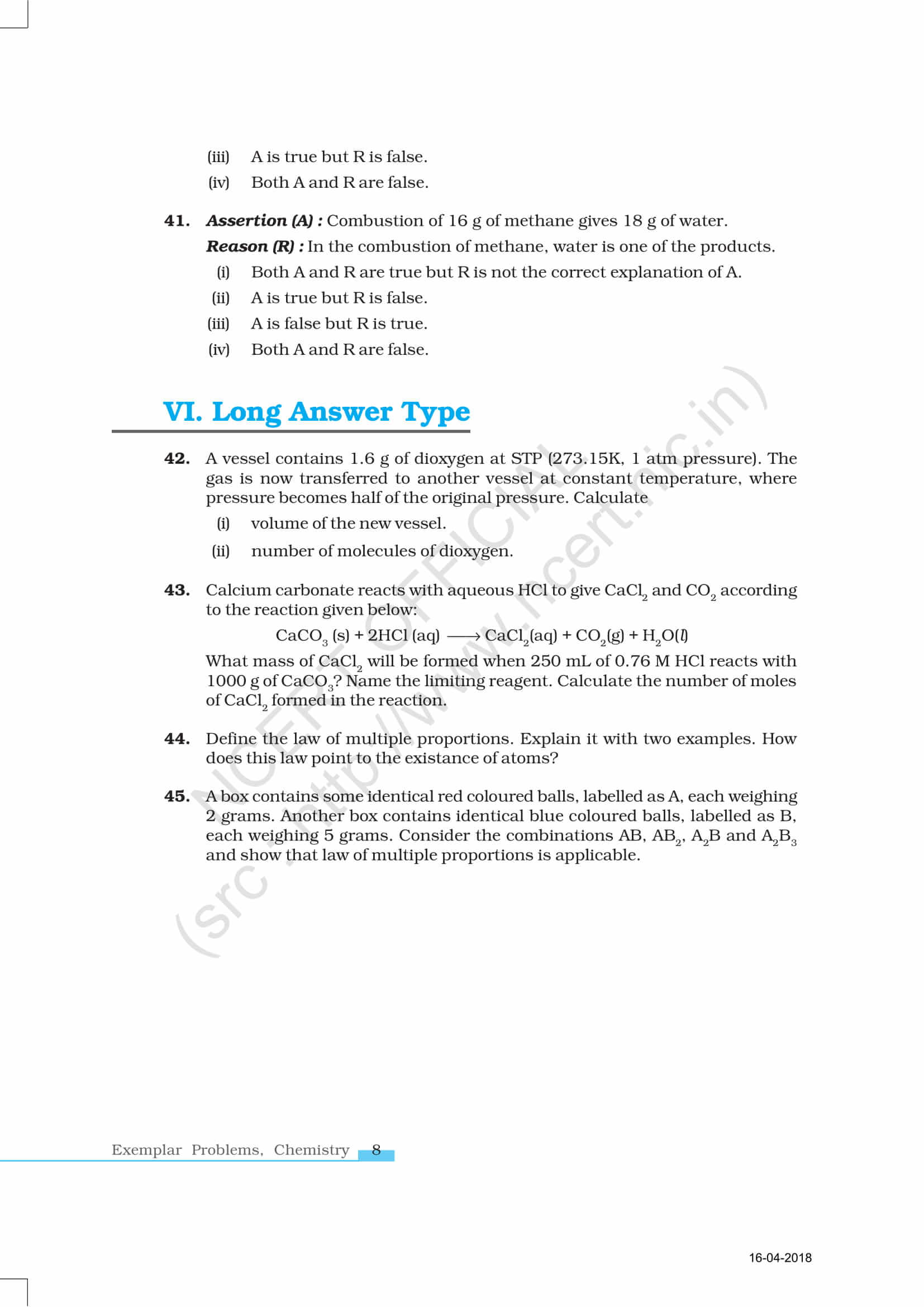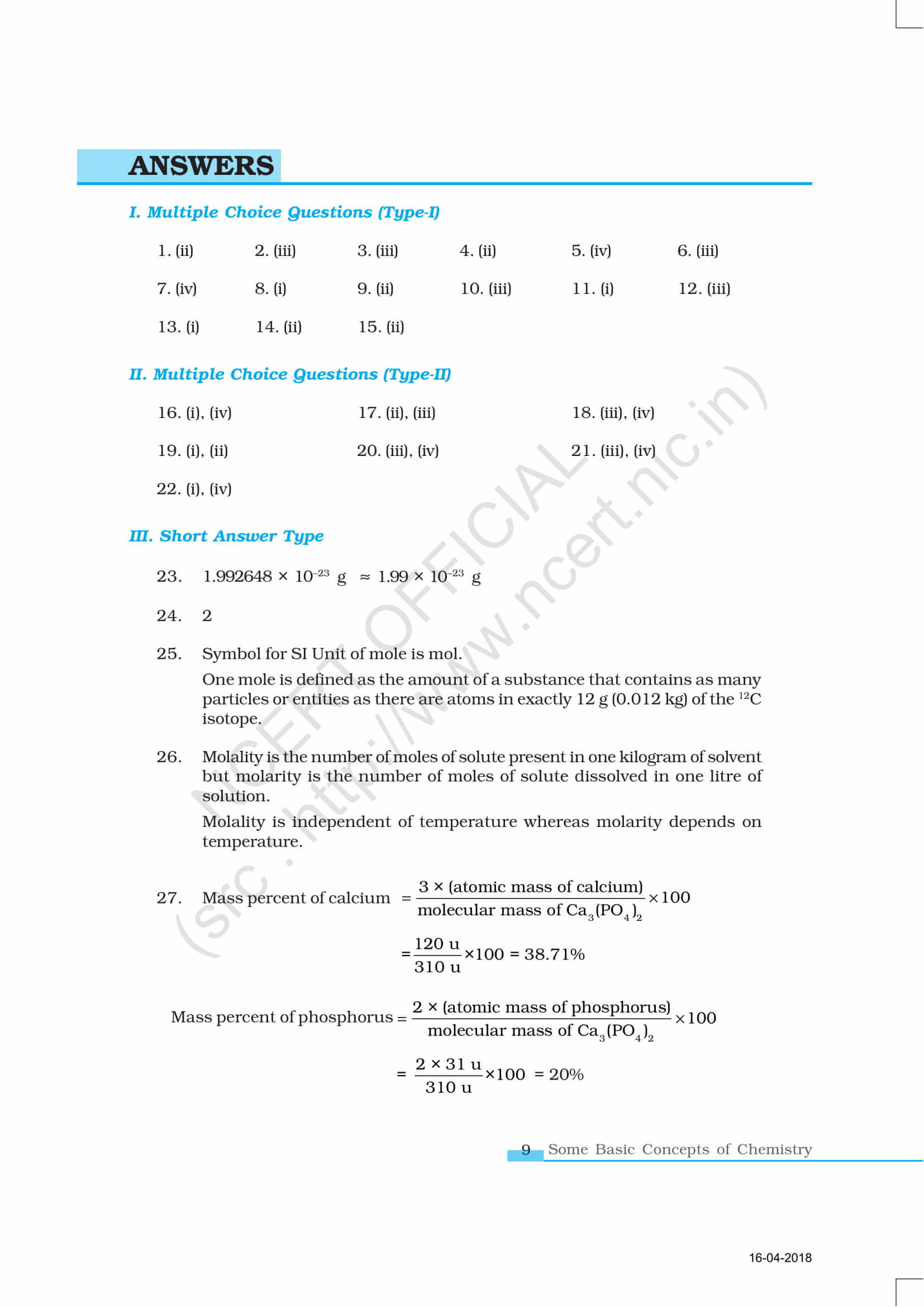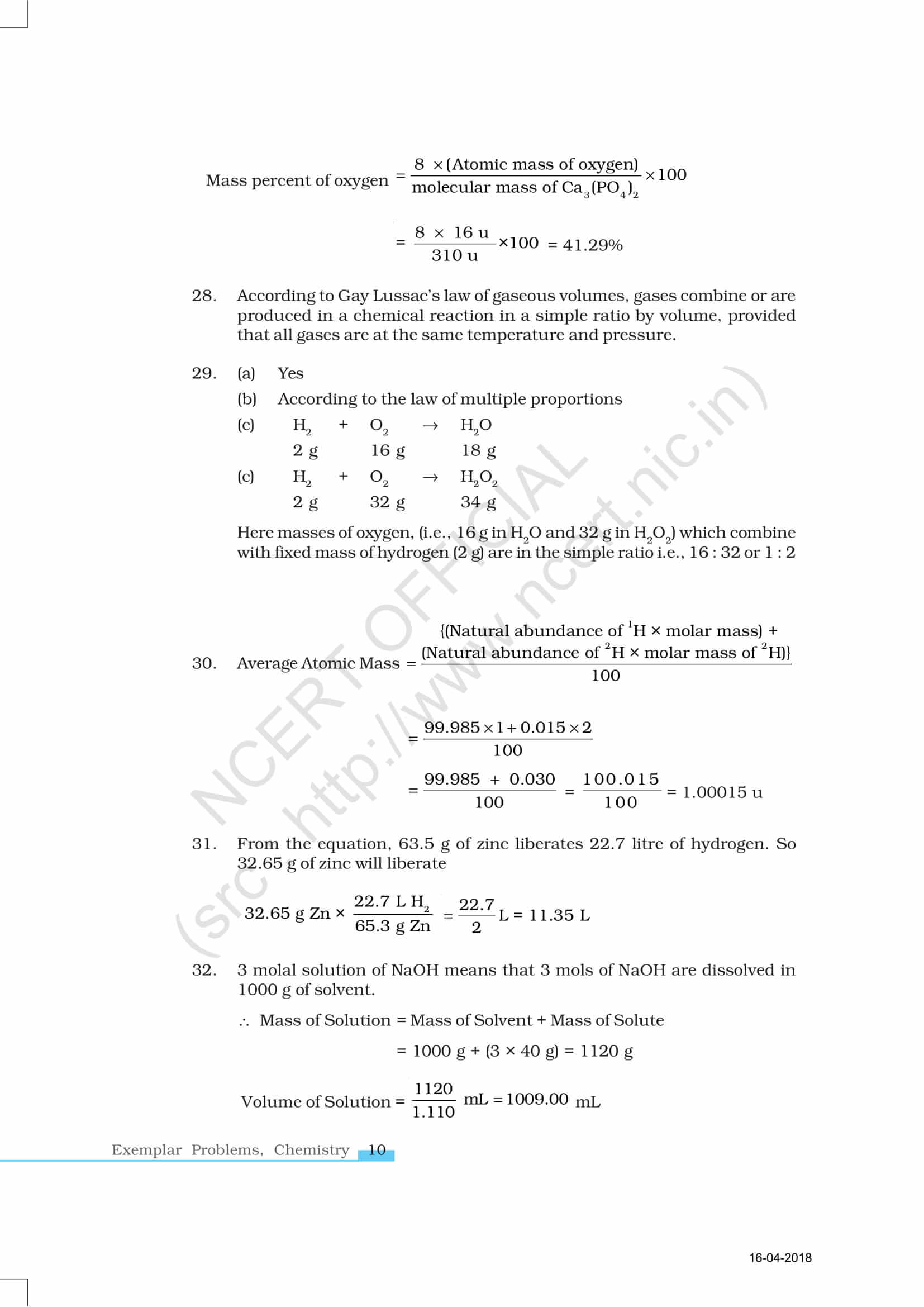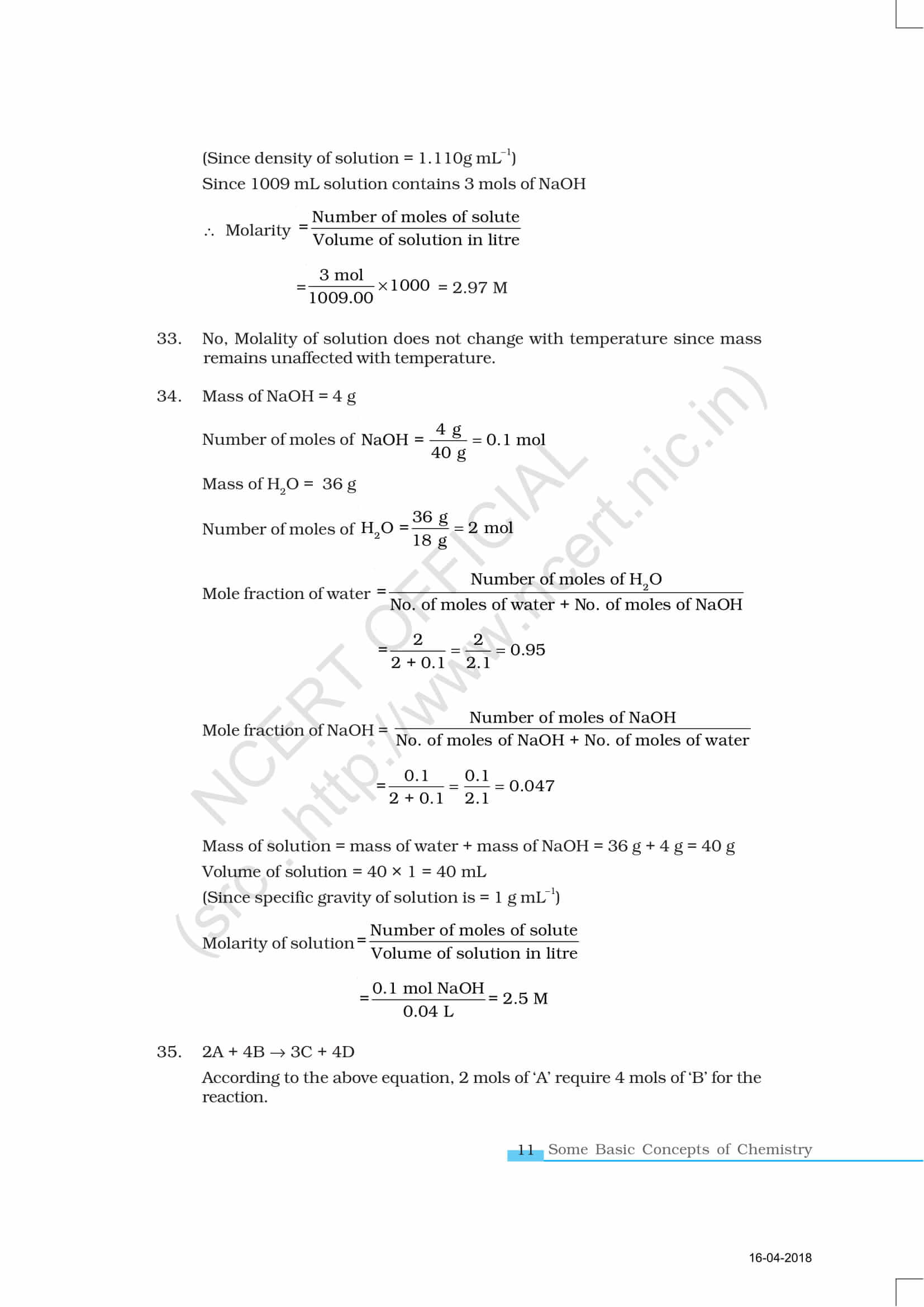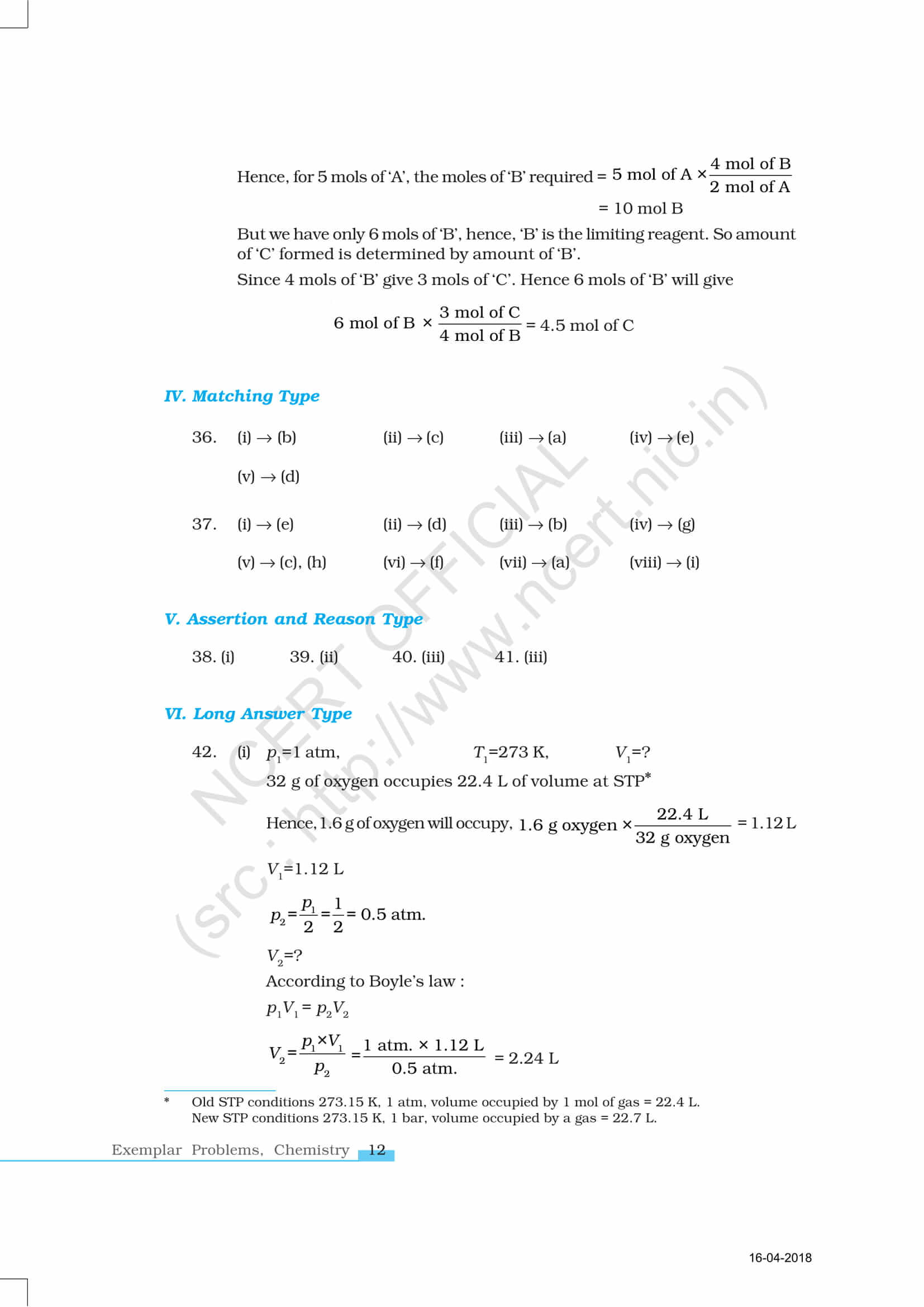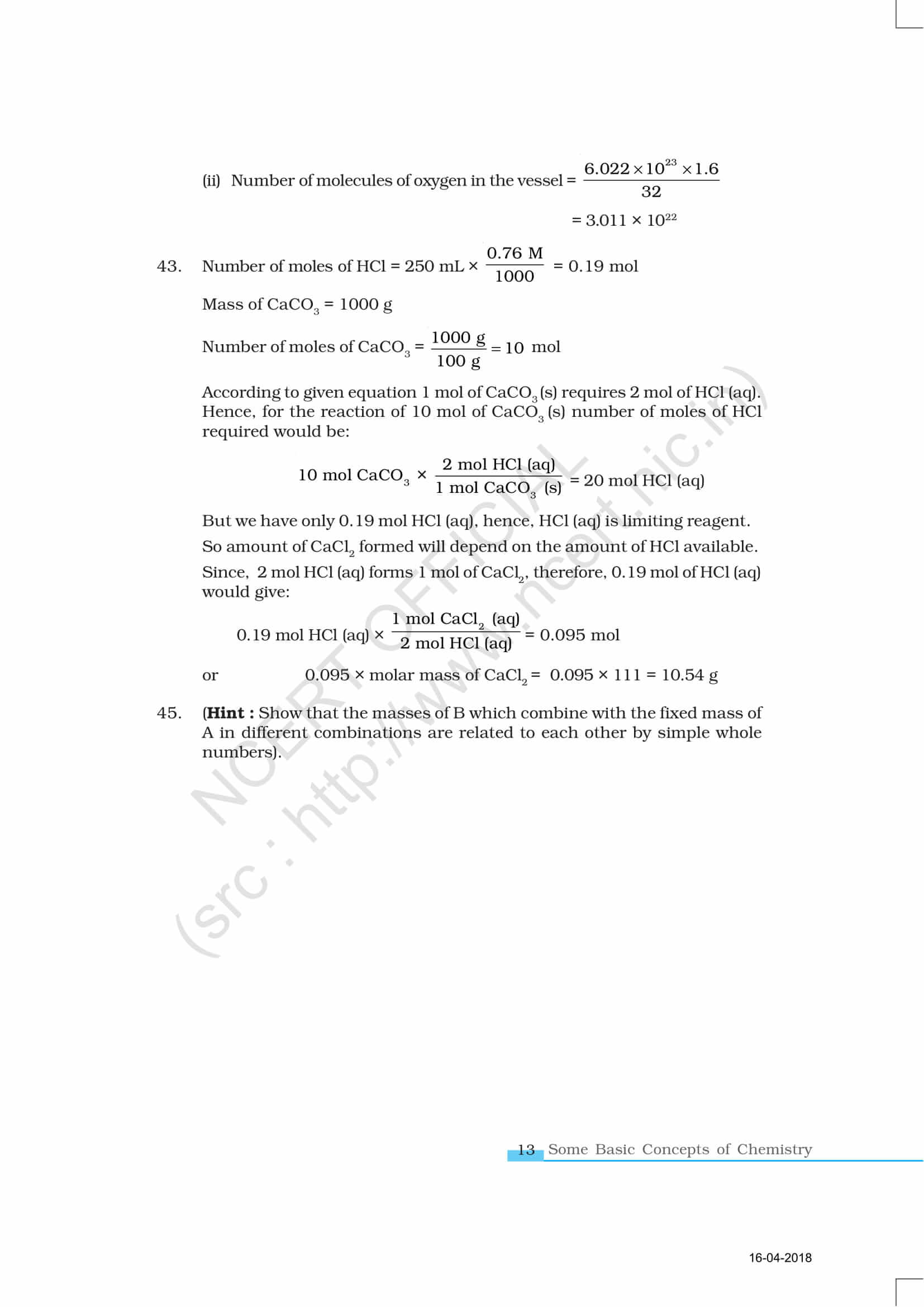At BYJU’S, students are provided with sample papers, previous year question papers, notes, exemplars, study materials, exercises, worksheets, tips and tricks to help them prepare for their class 11 exam and entrance exams in a more effective way.